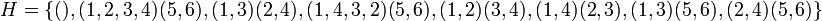D8 in A6

This article is about a particular subgroup in a group, up to equivalence of subgroups (i.e., an isomorphism of groups that induces the corresponding isomorphism of subgroups). The subgroup is (up to isomorphism) dihedral group:D8 and the group is (up to isomorphism) alternating group:A6 (see subgroup structure of alternating group:A6).
VIEW: Group-subgroup pairs with the same subgroup part | Group-subgroup pairs with the same group part | All pages on particular subgroups in groups

This article is about the subgroup$H$ in the group$G$, where$G$ is alternating group:A6, i.e., the alternating group on the set$\{ 1,2,3,4,5,6 \}$, and$H$ is the subgroup:$\! H = \{ (), (1,2,3,4)(5,6), (1,3)(2,4), (1,4,3,2)(5,6), (1,2)(3,4), (1,4)(2,3), (1,3)(5,6), (2,4)(5,6) \}$$H$ is a 2-Sylow subgroup of$G$ and is isomorphic to dihedral group:D8. There is a total of 45 conjugate subgroups to$H$ in$G$ (including$H$ itself).

Arithmetic functions

Function Value Explanation Comment
order of the group 360
order of the subgroup 8
index of the subgroup 45
size of conjugacy class 45
number of conjugacy classes in automorphism class 1

Subgroup properties

Resemblance-based properties

Property Meaning Satisfied? Explanation Comment
order-conjugate subgroup conjugate to any subgroup of the same order Yes Sylow implies order-conjugate
order-dominating subgroup any subgroup of the whole group whose order divides the order of$H$ is contained in a conjugate of$H$ Yes Sylow implies order-dominating
order-dominated subgroup any subgroup of the whole group whose order is a multiple of the order of$H$ contains a conjugate of$H$ Yes Sylow implies order-dominated
order-isomorphic subgroup isomorphic to any subgroup of the group of the same order Yes (via order-conjugate)
isomorph-automorphic subgroup Yes (via order-conjugate)
automorph-conjugate subgroup Yes (via order-conjugate)
order-automorphic subgroup Yes (via order-conjugate)
isomorph-conjugate subgroup Yes (via order-conjugate)

Normality-related properties

Property Meaning Satisfied? Explanation Comment
normal subgroup equals all its conjugate subgroups No (see other conjugate subgroups)
subnormal subgroup No
self-normalizing subgroup equals its normalizer in the whole group Yes
abnormal subgroup Yes
weakly abnormal subgroup Yes
contranormal subgroup Yes
maximal subgroup Yes
pronormal subgroup Yes Sylow implies pronormal
weakly pronormal subgroup Yes (via pronormal)

Fusion system

The subgroup embedding induces the simple fusion system for dihedral group:D8.

GAP implementation

Construction of subgroup given group as a black box

Suppose we are already given a group$G$ that we know to be isomorphic to alternating group:A6. Then, the subgroup$H$ can be constructed using SylowSubgroup as follows:

H := SylowSubgroup(G,2);

Construction of group-subgroup pair

The group and subgroup pair can be defined using GAP's AlternatingGroup and SylowSubgroup functions as follows:

G := AlternatingGroup(6); H := SylowSubgroup(G,2);